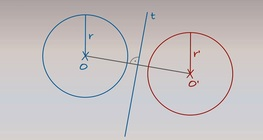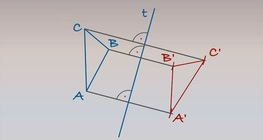Quantity: 0

Total: 0,00

# Reflecting a line segment across an axis

### Reflecting a line segment across an axis

Axis t and line segment AB are given on a plane. Let’s plot the mirror image of line segment AB across t.

02:16

Mathematics

Keywords

editing, geometry, reflection, section, mathematics

Related items

### On-screen labels

Reflecting a line segment across an axis, sketch:

### Related items

Information Reflecting a circle across an axis#### Reflecting a circle across an axis

Axis t and a circle with centre O and radius r are given on a particular plane. Let’s plot the mirror image of the circle.

Information Reflecting a triangle across an axis#### Reflecting a triangle across an axis

Axis t and triangle ABC are given on a particular plane. Let’s plot the mirror image of triangle ABC.